×#### Thank you for registering.

One of our academic counsellors will contact you within 1 working day.

Click to Chat

1800-1023-196

+91-120-4616500

CART 0

• 0

MY CART (5)

Use Coupon: CART20 and get 20% off on all online Study Material

ITEM
DETAILS
MRP
DISCOUNT
FINAL PRICE
Total Price: Rs.

There are no items in this cart.
Continue Shopping• Complete JEE Main/Advanced Course and Test Series
• OFFERED PRICE: Rs. 15,900
• View Details

```Chapter 2: Polynomials Exercise – 2.1

Question: 1

Find the zeroes of each of the following quadratic polynomials and verify the relationship between the zeroes and their coefficients:

(i)  f(x) = x2 – 2x – 8

(ii)  g(s) = 4s2 – 4s + 1

(iii)  6x2 – 3 – 7x

(iv)  h(t) = t2 – 15

(v) p(x) = x2 + 2√2 x – 6

(vi) q(x) = √3 x2 + 10x + 7√3

(vii) f(x) = x2 - (√3 + 1)x + √3

(viii)  g(x) = a(x2 + 1) – x(a2 + 1)

Solution:

(i) f(x) = x2 – 2x – 8

We have,

f(x) = x2 – 2x – 8

=  x2 – 4x + 2x – 8

=  x (x – 4) + 2(x – 4)

=  (x + 2)(x – 4)

Zeroes of the polynomials are – 2 and 4.

Now,Hence, the relationship is verified.

(ii)  g(s) = 4s2 – 4s + 1

We have,

g(s) = 4s2 – 4s + 1

= 4s2 – 2s – 2s + 1

= 2s(2s – 1)− 1(2s – 1)

= (2s – 1)(2s – 1)

Zeroes of the polynomials are 1/2 and 1/2.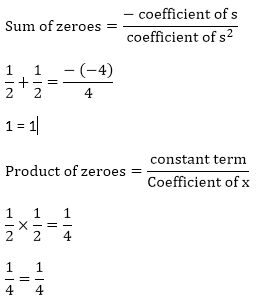Hence, the relationship is verified.

(iii) 6s2 − 3 − 7x

= 6s2 − 7x − 3 = (3x + 11) (2x – 3)

Zeros of the polynomials are 3/2 and (-1)/3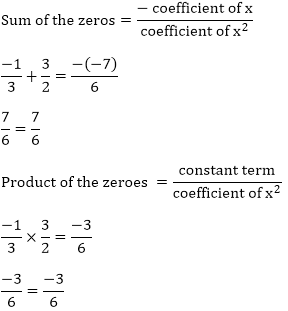Hence, the relationship is verified.

(iv) h(t) = t2 – 15

We have,Zeroes of the polynomials are - √15 and √15

Sum of the zeroes = 0 - √15 + √15 = 0

0 = 0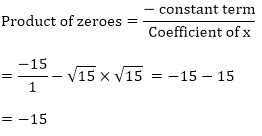Hence, the relationship verified.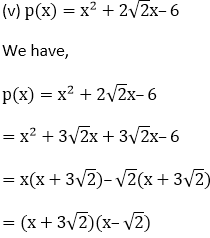Zeroes of the polynomials are 3√2 and –3√2 Sum of the zeroes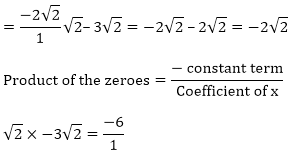-  6 = – 6

Hence, the relationship is verified.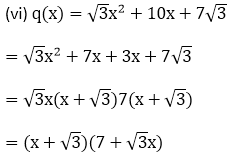Zeros of the polynomials are -√3 and -7/√3Product of the polynomials are - √3, 7/√3

7 = 7

Hence, the relationship is verified.Zeros of the polynomials are 1 and √3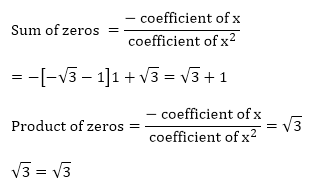Hence, the relationship is verified

(viii) g(x) = a[(x2 + 1)–  x(a2 + 1)]2

= ax2 + a − a2x − x

= ax2 − [(a2x + 1)] + a

= ax2 − a2x – x + a

= ax(x − a) − 1(x – a) = (x – a)(ax – 1)

Zeros of the polynomials are 1/a and 1 Sum of the zeros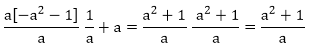Product of zeros = a/a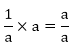Hence, the relationship is verified.

Question: 2

If α and β are the zeroes of the quadratic polynomial f(x) = x2 – 5x + 4, find the value of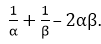Solution:

We have,

α and β are the roots of the quadratic polynomial.

f(x) = x2 – 5x + 4

Sum of the roots = α + β = 5

Product of the roots = αβ = 4

So,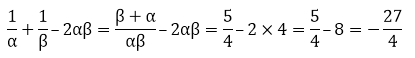Question: 3

If α and β are the zeroes of the quadratic polynomial f(x) = x2 – 5x + 4, find the value ofSolution:

Since, α and β are the zeroes of the quadratic polynomial.

p(y) = x2 – 5x + 4

Sum of the zeroes = α + β = 5

Product of the roots = αβ = 4

So,Question: 4

If α and β are the zeroes of the quadratic polynomial p (y) = 5y2 – 7y + 1, find the value of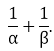Solution:

Since, α and β are the zeroes of the quadratic polynomial.

p(y) = 5y2 – 7y + 1

Sum of the zeroes = α + β = 7

Product of the roots = αβ = 1

So,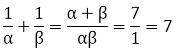Question: 5

If α and β are the zeroes of the quadratic polynomial f(x) = x2 – x – 4, find the value of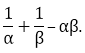Solution:

Since, α and β are the zeroes of the quadratic polynomial.

We have,

f(x) = x2 – x – 4

Sum of zeroes = α + β = 1

Product of the zeroes = αβ = - 4

So,Question: 6

If α and β are the zeroes of the quadratic polynomial f(x) = x2 + x – 2, find the value of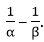Solution:

Since, α and β are the zeroes of the quadratic polynomial.

We have,

f(x) = x2 + x – 2

Sum of zeroes = α + β = 1

Product of the zeroes = αβ = – 2

So,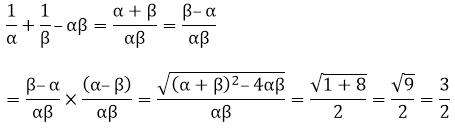Question: 7

If one of the zero of the quadratic polynomial f(x) = 4x2 – 8kx – 9 is negative of the other, then find the value of k.

Solution:

Let, the two zeroes of the polynomial f(x) = 4x2 – 8kx – 9 be α and − α.

Product of the zeroes = α × − α = – 9

Sum of the zeroes = α + (− α) = – 8k = 0                Since, α – α = 0

⇒ 8k = 0 ⇒ k = 0

Question: 8

If the sum of the zeroes of the quadratic polynomial f(t) = kt2 + 2t + 3k is equal to their product, then find the value of k.

Solution:

Let the two zeroes of the polynomial f(t) = kt2 + 2t + 3k be α and β.

Sum of the zeroes = α + β = 2

Product of the zeroes = α × β = 3k

Now,Question: 9

If α and β are the zeroes of the quadratic polynomial p(x) = 4x2 – 5x – 1, find the value of α2β + αβ2.

Solution:

Since, α and β are the zeroes of the quadratic polynomial p(x) = 4x2 – 5x – 1

So, Sum of the zeroes α + β = 5/4

Product of the zeroes  α × β = – 1/4

Now,

α2β + αβ2 = αβ (α + β)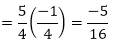Question: 10

If α and β are the zeroes of the quadratic polynomial

f(t) = t2 – 4t + 3, find the value of α4β3 + α3β4.

Solution:

Since, α and β are the zeroes of the quadratic polynomial f(t) = t2 – 4t + 3

So, Sum of the zeroes = α + β = 4

Product of the zeroes = α × β = 3

Now,

α4β3 + α3β4 = α3β3(α + β)

= (3)3(4) = 108

Question: 11

If α and β are the zeroes of the quadratic polynomial

f(x) = 6x2 + x – 2, find the value ofSolution:

Since, α and β are the zeroes of the quadratic polynomial f(x) = 6x2 + x – 2.

Sum of the zeroes = α + β = -1/6

Product of the zeroes =α × β = -1/3

Now,By substitution the values of the sum of zeroes and products of the zeroes, we will get

= - 25/12

Question: 12

If α and β are the zeroes of the quadratic polynomial f(x) = 6x2 + x – 2, find the value of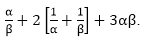Solution:

Since, α and β are the zeroes of the quadratic polynomial f(x) = 6x2 + x – 2.

Sum of the zeroes = α + β = 6/3

Product of the zeroes = α × β = 4/3

Now,By substituting the values of sum and product of the zeroes, we will get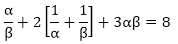Question: 13

If the squared difference of the zeroes of the quadratic polynomial

f(x) = x2 + px + 45 is equal to 144, find the value of p.

Solution:

Let the two zeroes of the polynomial be αand β.

We have,

f(x) = x2 + px + 45

Now,

Sum of the zeroes =  α + β = – p

Product of the zeroes =  α × β = 45

So,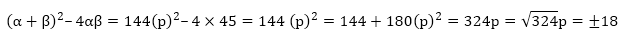Thus, in the given equation, p will be either 18 or -18.

Question: 14

If α and β are the zeroes of the quadratic polynomial

f(x) = x2 – px + q,  prove thatSolution:

Since, α and β are the roots of the quadratic polynomial given in the question.

f(x) = x2 – px + q

Now,

Sum of the zeroes = p = α + β

Product of the zeroes = q = α × β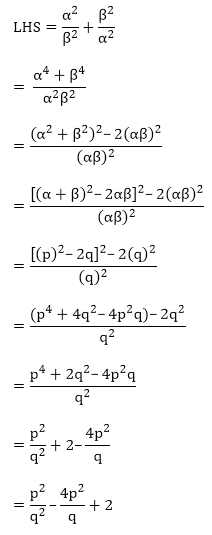LHS = RHS

Hence, proved.

Question: 15

If α and β are the zeroes of the quadratic polynomial f(x) = x2 – p(x + 1) – c, show that (α + 1)(β + 1) = 1 – c.

Solution:

Since, α and β are the zeroes of the quadratic polynomial

f(x) = x2 – p(x + 1)– c

Now,

Sum of the zeroes = α + β = p

Product of the zeroes = α × β = (- p – c)

So,

(α + 1)(β + 1)

= αβ + α + β + 1

= αβ + (α + β) + 1

= (− p – c) + p + 1

= 1 – c = RHS

So, LHS = RHS

Hence, proved.

Question: 16

If α and β are the zeroes of the quadratic polynomial such that α + β = 24 and α – β = 8, find a quadratic polynomial having α and β as its zeroes.

Solution:

We have,

α + β = 24         …… E-1

α – β = 8              …. E-2

By solving the above two equations accordingly, we will get

2α = 32 α = 16

Substitute the value of α, in any of the equation. Let we substitute it in E-2, we will get

β = 16 – 8 β = 8

Now,

Sum of the zeroes of the new polynomial = α + β = 16 + 8 = 24

Product of the zeroes = αβ = 16 × 8 = 128

x2– (sum of the zeroes)x + (product of the zeroes) =  x2 – 24x + 128

Hence, the required quadratic polynomial is f(x) = x2 + 24x + 128

Question: 17

If α and β are the zeroes of the quadratic polynomial

f(x) = x2 – 1, find a quadratic polynomial whose zeroes are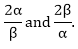Solution:

We have,

f(x) = x2 – 1

Sum of the zeroes = α + β = 0

Product of the zeroes = αβ = – 1

From the question,

Sum of the zeroes of the new polynomial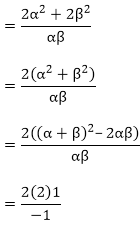{By substituting the value of the sum and products of the zeroes}

As given in the question,

Product of the zeroes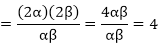x2 – (sum of the zeroes)x + (product of the zeroes)

= kx2 – (−4)x + 4x2 –(−4)x + 4

Hence, the required quadratic polynomial is f(x) = x2 + 4x + 4

Question: 18

If α and β are the zeroes of the quadratic polynomial f(x) = x2 – 3x – 2, find a quadratic polynomial whose zeroes are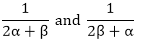Solution:

We have,

f(x) = x2 – 3x – 2

Sum of the zeroes =  α + β = 3

Product of the zeroes = αβ = – 2

From the question,

Sum of the zeroes of the new polynomial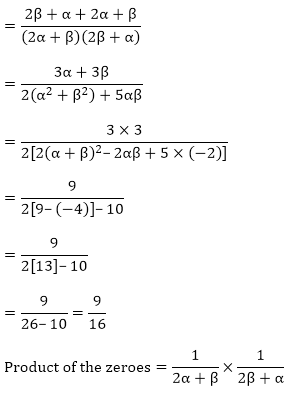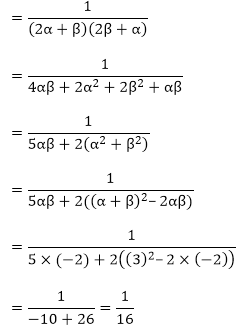x2- (sum of the zeroes)x + (product of the zeroes)

x2 - (sum of the zeroes)x + (product of the zeroes)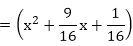Hence, the required quadratic polynomial is kQuestion: 19

If α and β are the zeroes of the quadratic polynomial f(x) = x2 + px + q, form a polynomial whose zeroes are (α + β)2 and (α – β)2.

Solution:

We have,

f(x) = x2 + px + q

Sum of the zeroes = α + β = -p

Product of the zeroes = αβ = q

From the question,

Sum of the zeroes of new polynomial = (α + β)2 + (α – β)2

= (α + β)2 + α2 + β2 – 2αβ

= (α + β)2 + (α + β)2 – 2αβ – 2αβ

= (- p)2 + (- p)2 – 2 × q – 2 × q

= p2 + p2 – 4q

= p2 – 4q

Product of the zeroes of new polynomial = (α + β)2 (α – β)2

= (- p)2((- p)2 - 4q)

= p2 (p2–4q)

x2 – (sum of the zeroes)x + (product of the zeroes)

= x2 – (2p2 – 4q)x + p2(p2 – 4q)

Hence, the required quadratic polynomial is f(x) = k(x2 – (2p2 –4q) x + p2(p2 - 4q)).

Question: 20

If α and β are the zeroes of the quadratic polynomial f(x) = x2 – 2x + 3, find a polynomial whose roots are:

(i)  α + 2,β + 2Solution:

We have,

f(x) = x2 – 2x + 3

Sum of the zeroes = α + β = 2

Product of the zeroes = αβ = 3

(i) Sum of the zeroes of new polynomial = (α + 2) + (β + 2)

= α + β + 4

= 2 + 4 = 6

Product of the zeroes of new polynomial = (α + 1)(β + 1)

= αβ + 2α + 2β + 4

= αβ + 2(α + β) + 4 = 3 + 2(2) + 4 = 11

x2 – (sum of the zeroes)x + (product of the zeroes)

= x2 – 6x +11

Hence, the required quadratic polynomial is f(x) = k(x2 – 6x + 11)

f(x) = k(x2 – 6x + 11)

(ii) Sum of the zeroes of new polynomial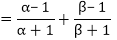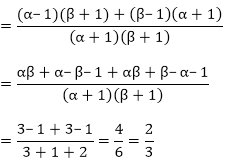Product of the zeroes of new polynomialx2 – (sum of the zeroes)x + (product of the zeroes)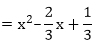Thus, the required quadratic polynomial is f(x) = k(x2 – 23x + 13)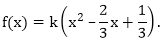Question: 21

If α and β are the zeroes of the quadratic polynomial f(x) = ax2 + bx + c, then evaluate:

(i)  α – β(iv) α2β + αβ2

(v) α4 + β4Solution:

f(x) = ax2 + bx + c

Here,

Sum of the zeroes of polynomial = α + β = -b/a

Product of zeroes of polynomial = αβ = c/a

Since, α + β are the roots (or) zeroes of the given polynomial, so

(i) α – β

The two zeroes of the polynomials are -From previous question we know that,Also,

αβ = c/a

Putting the values in E.1, we will get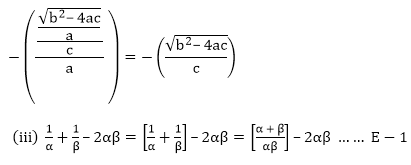Since,

Sum of the zeroes of polynomial = α + β = – b/a

Product of zeroes of polynomial = αβ = c/a

After substituting it in E-1, we will get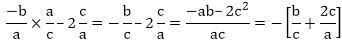(iv) α2β + αβ2

= αβ(α + β) …….. E- 1.

Since,

Sum of the zeroes of polynomial = α + β = – b/ a

Product of zeroes of polynomial = αβ = c/a

After substituting it in E-1, we will get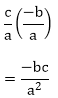(v)  α4 + β4

= (α2 + β2)2 – 2α2β2

= ((α + β)2 – 2αβ)2 – (2αβ)2 ……. E- 1

Since,

Sum of the zeroes of polynomial = α + β = – b/a

Product of zeroes of polynomial = αβ = c/a

After substituting it in E-1, we will get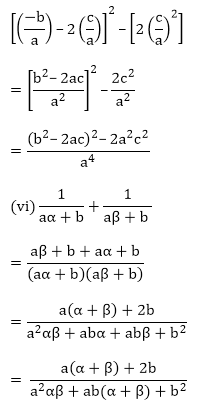Since,

Sum of the zeroes of polynomial = α + β = – b/a

Product of zeroes of polynomial = αβ = c/a

After substituting it, we will get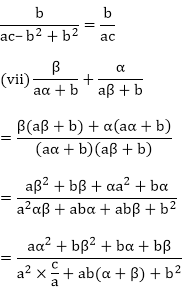Since,

Sum of the zeroes of polynomial = α + β = – b/a

Product of zeroes of polynomial = αβ = c/a

After substituting it, we will get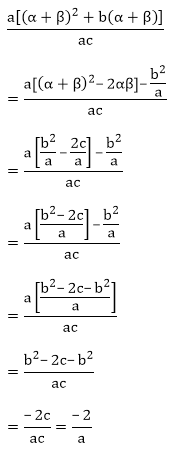Since,

Sum of the zeroes of polynomial= α + β = – b/a

Product of zeroes of polynomial= αβ = c/a

After substituting it, we will get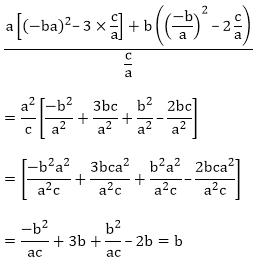```### Course Features

• 728 Video Lectures
• Revision Notes
• Previous Year Papers
• Mind Map
• Study Planner
• NCERT Solutions
• Discussion Forum
• Test paper with Video Solution